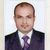# QlikView App Dev

Discussion Board for collaboration related to QlikView App Development.

Announcements
Our next Qlik Insider session will cover new key capabilities. Join us August 11th REGISTER TODAY
cancel
Showing results for
Did you mean:Not applicable

## Trend line showing the combination of data?

Hi,

I have two Line charts.

Chart A have

Dimension: Band

Expression: Sum(Number of Cases)

Chart B Have

Dimension: Band

Expression: Sum(Cases)

I want to combine the two charts. I need to show the trend line of sum of Cases when compared to last week and current week.

Can anyone suggest me please how to set this.

Thanks.

12 RepliesPartner

I set the trendlines only for the differenceNot applicable
Author

Thanks. seems found the solution But I will let you know if this is not my user needs?MVP

Create a single chart

Dimension:- Band

Expression for 15042014:- Sum({< Date = {'\$(=date( WeekStart(Today())-6))'}>} [Number of Cases])

Expression for 23042014:- Sum({< Date = {'\$(=Today()))'}>} Cases)

Expression Difference:- Sum({< Date = {'\$(=date( WeekStart(Today())-6))'}>} [Number of Cases]) -

Sum({< Date = {'\$(=Today()))'}>} Cases)Community Browser